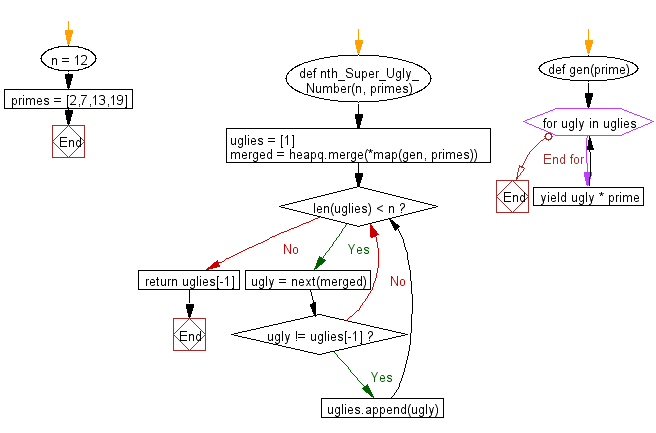﻿ Python heap queue algorithm: Find the nth super ugly number from a given prime list of size k using Heap queue algorithm - w3resource

# Python: Find the nth super ugly number from a given prime list of size k using Heap queue algorithm

## Python heap queue algorithm: Exercise-13 with Solution

Write a Python program to find the nth super ugly number from a given prime list of size k using the heap queue algorithm.

Super ugly numbers are positive numbers whose all prime factors are in the given prime list primes of size k. For example, [1, 2, 4, 7, 8, 13, 14, 16, 19, 26, 28, 32] is the sequence of the first 12 super ugly numbers given primes = [2, 7, 13, 19] of size 4.

Sample Solution:

Python Code:

``````import heapq
#Ref.: https://bit.ly/32c9P3A
def nth_Super_Ugly_Number(n, primes):
uglies = 

def gen(prime):
for ugly in uglies:
yield ugly * prime

merged = heapq.merge(*map(gen, primes))
while len(uglies) < n:
ugly = next(merged)
if ugly != uglies[-1]:
uglies.append(ugly)
return uglies[-1]
n = 12
primes = [2,7,13,19]
print(nth_Super_Ugly_Number(n, primes))
```
```

Sample Output:

```32
```

Flowchart:Python Code Editor:

Have another way to solve this solution? Contribute your code (and comments) through Disqus.

What is the difficulty level of this exercise?

Test your Programming skills with w3resource's quiz.

﻿